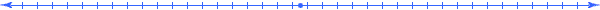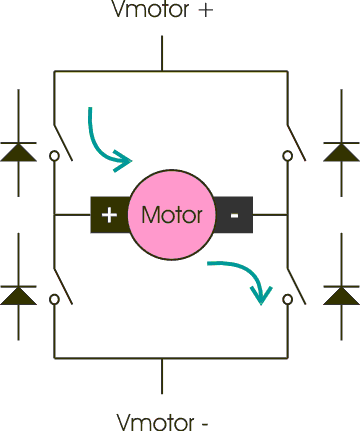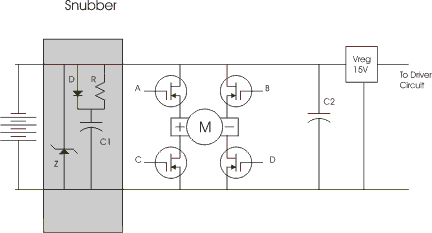# Voltage Spikes in FET based H-bridgesBy: Chuck McManis May 20, 2002

## Overview

I had a problem, a big one. My brand new speed controller design was causing my cool HP power supply to shut down. The symptoms were that when a serious load spun up, and then slowed down, the HP shut down with the OVP (over voltage protection) light turned on. Note that this is different than the CC light which says the supply is limiting current and voltage is suffering.

## Hypothesis

So taking what I knew, I tried to construct a hypothesis for why this was occurring, and then come up with some sort of experiment that would prove or disprove that hypothesis.

A simplified view of the H-bridge is shown below. There are four logical switches (upper left, lower left, upper right, and lower right) that can be turned on or off. Because they are implemented with MOSFETs, there is a natural parasitic diode that is reverse biased across each switch.The diagram shows the flow of current (green arrows) when the upper left and lower right switches are turned "on".

The controller uses Pulse Width Modulation (PWM) to control how much current flows through the motor. It attempts to limit current "rushes" by ramping the current from zero (motor not operating) to 100%, then on the change of direction from 100% to 100% the other direction.

The interesting bit comes when the controller is asked to stop the motor. This occurs when it receives a signal indicating zero speed (neutral pulse from the R/C receiver).

When the controller is commanded to stop, it first slews back from what it is currently doing to 0%, then it slews from 0% to 100% "braking." Braking is accomplished by turning on both lower switches. This creates a current path between the terminals of the motor, and if the motor is turning the motor will generate a voltage resists the motors turning direction. (This is called back EMF in the motor tutorial).  This back EMF then slows the motor down until the motor stops.

Braking, like going forward and backward, starts out with a 0% duty cycle PWM wave and then slews up to 100% duty cycle. This starts braking softly and then increases up to a full brake condition. The current flow is shown in the diagram below.As you can there is a current loop in the picture and a diagram above showing the voltage output (the dotted line represents ground).

The hypothesis was that by shorting the motor, I induced a change in the current flowing through the motor, this change in current was reflected as a voltage potential across the motor terminals and was governed by the equation

V = L di/dt                            1.0

The equation is the classic description of voltage behavior across a coil due to a change in current.

The perplexing part to me was how could the voltage be greater than the input voltage? The theory is that by rapidly changing the current I induced a higher voltage spike than simply the back EMF voltage.

## Experiment

To prove or disprove this hypothesis, I needed to be able to change the induced voltage at will. To do that I need to change either di (the change in current) or dt (the rate at which it changed).

The braking code in my speed controller consists of adjusting the PWM duty cycle to bring it closer to the final desired value every 10mS.

Thus to change dt, I needed to change the number of "ticks" it took to go from 0 to 100% braking. I could go from 0 to full brake in one cycle making dt 10ms. Or I could adjust it by 10% each time going from 0 to full brake in 10 steps making dt 100ms, etc.

To change di I needed to change the current that was seen by the circuit. Clearly I do that in the drive circuit today by changing the PWM width. The closer I get to 100% duty cycle, the more current I'm dumping through the motors. Thus by setting the "maximum" PWM value to something less than 100% I could control the change in current

## Results

The results were fairly conclusive. Using "the HP supply turns off" as the test condition. I found that by reducing the braking time to 600mS the HP would not see any over voltage condition. If I left the braking rate alone and changed the PWM duty cycle of the braking to < 15% then I was also "safe" in terms of voltage spikes.

### Snubber Design

This is the snubber I used on the working speed controller## Follow Up

As it turns out I was close but not exactly correct. After reading up on snubbers in the book "Power Electronics" [POWER1] I discovered I had solved the problem.

There are three types of snubbers;

• Turn-on snubbers that control the turn on "trajectory",

• Turn-off snubbers that absorb inductive kicks,

• and Over voltage snubbers that deal with voltage kicks from wiring inductance.

Yes, I said "wiring inductance."

The turn-off snubbers are the ones that would be across a FET and would help it deal with the voltage spike that occurs when the motor coil is de-energized. This is a problem but the body diode often is sufficient and a small RCD snubber can pick up any excess fairly easily. The tricker one is the over voltage snubber. Now in this one you see you've started pulling some number of amps of power from the power source (typically batteries) and suddenly you stop. Whoops! There is a small bit of inductance in the wiring between the batteries and the speed controller and that inductance suddenly does not want to have the current stop and so it presents a higher and higher voltage until somehow the current does manage to flow (typically though burned out FETs). Now with an RCD snubber here the capacitor absorbs that pulse, and when you next switch on the FETs you burn off what was absorbed in the capacitor in the resistor attached to the capacitor.

But how much capacitance do you need? Well if you consider the worst case to be the power supply delivering 400 amps that get suddenly "snapped off" then the di/dt term will be 400 over 150 nS or 2.6 x 10^9 so for each uH of inductance you have that would be a voltage change of 2.6 kV. Now assuming you've got a capacitor that can tolerate an inrush of 30 amps, you get 200 V per uF of capacitance and that means 200 uF of capacitance can absorb your pulse (if you let it suck 30A). But that has to be 200uF of "available" capacitance. And since charge = CV and you want the max voltage rise to be about 10V, that means you need roughly 10 times that much or 2,000 uF of "excess" capacitance.

--ChuckReturn to the Projects Page Return To Robotics Notebook